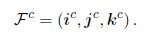## Estimation using camera data

This section introduces state estimation using camera data and the control of a gimbal. First, we address the coordinate frame geometry and describe the gimbal and camera frames. Then, the projection of a 3-D object into the 2-D image plane is presented. We also present a simple gimbal pointing algorithm which is implemented in a pan-tilt gimbal to point an antenna towards the UAS. Moreover, we discuss a geolocation algorithm used to estimate the position of an aircraft within its eld of view. This study assumes that an image recognition algorithm exists which detects the UAS in the image data. This section is
based on the work of.

## Gimbal and Camera Frames and Projective Geometry

Assuming that the center of mass of the antenna pointer is the origin of the gimbal and camera frames, there are three frames of interest. The camera frame denoted byThe second frame of interest is obtained by rotating the body frame an angle of, the azimuth angle, about theaxis, resulting in the gimbal frame (g1). This frame is denoted byThe rotation matrix of this coordinate frame transformation is given by15

Finally, if the gimbal-1 frame is rotated an angle of, the elevation angle, in theaxis, the third frame of interest is obtained, which is the gimbal frame. This transformation is described by16

To obtain the rotation matrix from the body frame to the gimbal frame we just need to multiply the matrices in 15 and 16, resulting in17

The convention in computer vision and image processing for the camera frame is that theaxis points to the right of the image,axis points down of the image, andaxis points along the optical axis. Therefore, the transformation from the gimbal frame to the camera frame is given by18

## Camera Model

Lets assume that the pixels and the pixel array are square. Dening M as- the width of the square pixel array and v as the eld-of-view of the camera, then f which is the focal length, is given by19

The position of the UAS projected into the camera frame is given by (), whereandare the pixel location of the aircraft in units of pixels. The distance between the origin of the camera frame and the location of the plane (), is PF whereUsing basic trigonometry we get21,22,23

Deningas the vector to the UAS and L = ||l|| we can express (21) through (23) as24

since L is unknown,cannot be calculated only with camera data. Even so, the unit vector that points towards the aircraft can be obtained as25

Since this unit vector is used multiple times throughout this section, we use the notation## Gimbal Pointing

In this section we present a basic gimbal-pointing algorithm, which is used by our antenna pointer. The tracking system is assumed to pan and tilt with the respective angles azimuth and elevation. The dynamic model of the gimbal is assumed asThe purpose of this algorithm is to point an antenna mounted in the gimbal to a given position, in our case the aircraft location. Letbe the position of the UAV in the inertial frame. Our goal is to center the aircraft in the image plane, therefore aligning the optical axis of the camera with the desired relative position vectorwhereis the inertial position of the antenna pointer and the subscript d indicates a desired quantity. The unit vector that targets the UAS in the body-frame isFurthermore, we need to calculate the desired azimuth and elevation angles so that the UAS is located in the origin of the image plane, this is, to align the optical axis with. The next step is to determine the desired azimuth and elevation angles that aligns the optical axis with. Since the optical axis is given by, the c denoting that is in the camera frame, the commanded gimbal anglesandare26,27,28

Therefore the desired azimuth and elevation angles are29,30

Then, choosing the gimbal servo commands we have31,32

whereandare positive control gains.